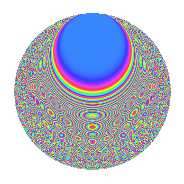# Properties

 Label 2541.2.eLevel 2541 Weight 2 Character orbit e Rep. character $$\chi_{2541}(1574,\cdot)$$ Character field $$\Q$$ Dimension 272 Sturm bound 704

# Related objects

## Defining parameters

 Level: $$N$$ = $$2541 = 3 \cdot 7 \cdot 11^{2}$$ Weight: $$k$$ = $$2$$ Character orbit: $$[\chi]$$ = 2541.e (of order $$2$$ and degree $$1$$) Character conductor: $$\operatorname{cond}(\chi)$$ = $$21$$ Character field: $$\Q$$ Sturm bound: $$704$$

## Dimensions

The following table gives the dimensions of various subspaces of $$M_{2}(2541, [\chi])$$.

Total New Old
Modular forms 376 308 68
Cusp forms 328 272 56
Eisenstein series 48 36 12

## Trace form

 $$272q - 248q^{4} + 8q^{7} + 8q^{9} + O(q^{10})$$ $$272q - 248q^{4} + 8q^{7} + 8q^{9} + 20q^{15} + 200q^{16} + 12q^{18} + 10q^{21} + 184q^{25} - 12q^{28} + 4q^{30} - 44q^{36} + 32q^{37} - 16q^{39} - 20q^{42} + 40q^{43} + 16q^{46} + 8q^{51} + 4q^{57} + 24q^{58} - 132q^{60} - 6q^{63} - 104q^{64} - 96q^{67} + 12q^{70} - 24q^{72} + 20q^{78} - 56q^{79} - 24q^{81} - 100q^{84} + 8q^{85} + 36q^{91} + 28q^{93} + O(q^{100})$$

## Decomposition of $$S_{2}^{\mathrm{new}}(2541, [\chi])$$ into newform subspaces

The newforms in this space have not yet been added to the LMFDB.

## Decomposition of $$S_{2}^{\mathrm{old}}(2541, [\chi])$$ into lower level spaces

$$S_{2}^{\mathrm{old}}(2541, [\chi]) \cong$$ $$S_{2}^{\mathrm{new}}(231, [\chi])$$$$^{\oplus 2}$$

## Hecke Characteristic Polynomials

There are no characteristic polynomials of Hecke operators in the database